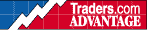HOT TOPICS LIST

INDICATORS LIST

LIST OF TOPICS

08/11/00 08:03:34 AM
by Dennis D. Peterson

Constructing an Excel spreadsheet requires that each step of the ADX computation be stated. Shown are each of the calculations.

Security:   NASDAQ Composite
Position:   N/A

 As promised, this posting gives the Excel spreadsheet formulas for the ADX calculations along with a more detailed explanation of the five possible ADX cases that can occur. This is reprinted in part from Volume 11, Issue 4,of the STOCKS & COMMODITIES article, "The ADX" by Thom Hartle.Figure 1 shows the five ADX cases that have to be resolved. In example A, the second day's trading range is higher than the first day's trading range, indicating positive directional movement. In example B, the second day's trading range is below the first day's trading range, an indication of negative directional movement. Example C is more complicated because the second day's range is both lower and higher than the first day's range. Directional movement is only considered to be up, down or not present. Therefore, the larger part of the day's range extending beyond the previous day's range is used to identify directional movement. In example C, the largest part of the second day is higher; consequently, the directional movement is positive. In example D, the largest part of the second day's range is lower so that the directional movement is negative. In example E, the second day's range is within the first day's range so the directional movement is zero. In the Excel 4.0 spreadsheet (Figure 2), the first calculation for ADX is the true range value (TR). This is performed in column E. The formula for cell E3 is:=MAX(B3-C3,ABS(B3-D2),ABS(C3-D2))Next, column F determines the positive directional movement or returns zero if there is no positive directional movement. The formula for cell F3 is:=IF(B3-B2>C2-C3,MAX(B3-B2,0),0)Column G calculates the negative directional movement or returns zero if there is no negative directional movement. The formula for cell G3 is:=IF(C2-C3>B3-B2,MAX(C2-C3,0),0)The daily calculations are volatile and so the data needs to be smoothed. First, sum the last 14 periods for TR, +DM and - DM. The formula for summing the TR is in cell H16:=SUM(E3:E16)Figure 2: ADX spreadsheet. Here is the output for the S&P 500 from January 1 to February 11, 1992. The formula for summing the +DM is in cell I16:=SUM(F3:F16)The formula for summing the -DM is in cell J16:=SUM(G3:G16)The smoothing formula for the TR14 column begins at cell H17:=TRUNC((H16-(H16/14)+E17),3)The smoothing formula subtracts 1/14th of yesterday's TR14 from yesterday's TR14 and then adds today's TR value. The truncating function is used to calculate the indicator as close as possible to the developer of the ADX's original form of calculation (which was done by hand). The smoothing formula for the +DM14 column begins at cell I17:=TRUNC((I16-(I16/14)+F17),3)The smoothing formula subtracts 1/14th of yesterday's +DM14 from yesterday's +DM14 and then adds today's +DM value. The smoothing formula for the -DM14 column begins at cell J17:=TRUNC((J16-(J16/14)+G17),3)The smoothing formula subtracts 1/14th of yesterday's -DM14 value from yesterday's -DM14 and then adds today's -DM value. Now we have a 14-day smoothed sum of TR, +DM and -DM. The next step is to calculate the ratios of +DM and -DM to TR. The ratios are called the +directional indicator (+DI) and -directional indicator (-DI). The formula for the +DI column begins at cell K16:=INT(100*(I16/H16)) The formula for the +DI column begins at cell L16:=INT(100*(J16/H16))The integer function (INT) is used because the original developer dropped the values after the decimal in the original work on the ADX indicator. The next step is to calculate the absolute value of the difference between the +DI and the -DI. This is done in column M and the formula for cell M16:=ABS(K16-L16)[Please note that in last week's article I needed to mention that DX is the ratio of the absolute value of the difference between +DI and -DI, since DX is positive.]The next column calculates the sum of the +DI and -DI. The formula for cell N16:=K16+L16The next step is to calculate the DX, which is the ratio of the absolute value of the difference between the +DI and the -DI divided by the sum of the +DI and the -DI. This is done in column O. The formula for cell O16:=INT(100*(M16/N16))The final step is smoothing the DX to arrive at the value of the ADX. First, average the last 14 days of DX values. The formula for cell P28:=AVERAGE(O15:O28)The smoothing process uses yesterday's ADX value multiplied by 13, and then add today's DX value. Finally, divide this sum by 14. The formula for cell P29:=INT(((P28*13)+O29)/14)Additional readingWilder, J. Welles . New Concepts in Technical Trading Systems, Trend Research.

Dennis D. Peterson

Market index trading on a daily basis.

 Comments or Questions? Article Usefulness 5 (most useful) 4 3 2 1 (least useful)

Date:�03/16/10Rank:�5Comment:�Thank you Mr. Dennis Peterson for this example. I have been able to follow it through have sucessfully embedded this model in my software. Great stuff. Kishor. Mistry
Date:�05/31/17Rank:�5Comment: